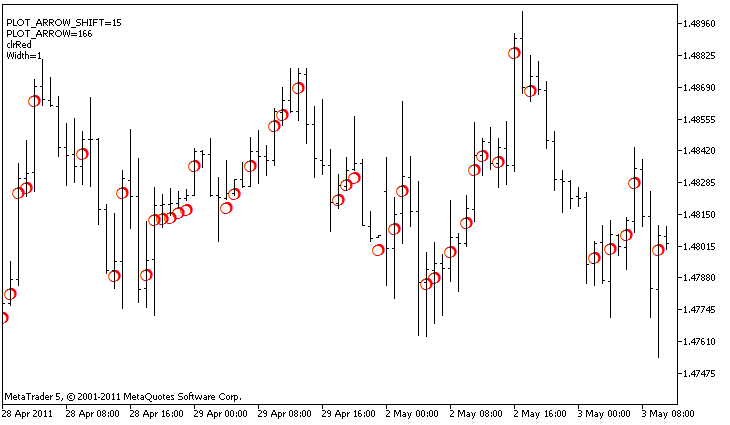# DRAW_ARROW

The DRAW_ARROW style draws arrows of the specified color (symbols of the set Wingdings) based on the value of the indicator buffer. The width and color of the symbols can be specified like for the DRAW_LINE style - using compiler directives or dynamically using the PlotIndexSetInteger() function. Dynamic changes of the plotting properties allows changing the look of an indicator based on the current situation.

The symbol code is set using the PLOT_ARROW property.

 //--- Define the symbol code from the Wingdings font to draw in PLOT_ARROW    PlotIndexSetInteger(0,PLOT_ARROW,code);

The default value of PLOT_ARROW=159 (a circle).

Each arrow is actually a symbol that has the height and the anchor point, and can cover some important information on a chart (for example, the closing price at the bar). Therefore, we can additionally specify the vertical shift in pixels, which does not depend on the scale of the chart. The arrows will be shifted down by the specified number of pixels, although the values of the indicator will remain the same:

 //--- Set the vertical shift of arrows in pixels    PlotIndexSetInteger(0,PLOT_ARROW_SHIFT,shift);

A negative value of PLOT_ARROW_SHIFT means the shift of arrows upwards, a positive values shifts the arrow down.

The DRAW_ARROW style can be used in a separate subwindow of a chart and in its main window. Empty values are not drawn and do not appear in the "Data Window", all the values in the indicator buffers should be set explicitly. Buffers are not initialized with a zero value.

 //--- Set an empty value    PlotIndexSetDouble(index_of_plot_DRAW_ARROW,PLOT_EMPTY_VALUE,0);

The number of buffers required for plotting DRAW_ARROW is 1.

An example of the indicator, which draws arrows on each bar with the close price higher than the close price of the previous bar. The color, width, shift and symbol code of all arrows are changed randomly every N ticks.In the example, for plot1 with the DRAW_ARROW style, the properties, color and size are specified using the compiler directive #property, and then in the OnCalculate() function the properties are set randomly. The N parameter is set in external parameters of the indicator for the possibility of manual configuration (the Parameters tab in the indicator's Properties window).

 //+------------------------------------------------------------------+ //|                                                   DRAW_ARROW.mq5 | //|                        Copyright 2011, MetaQuotes Software Corp. | //|                                              https://www.mql5.com | //+------------------------------------------------------------------+ #property copyright "Copyright 2011, MetaQuotes Software Corp." #property link      "https://www.mql5.com" #property version   "1.00"   #property description "An indicator to demonstrate DRAW_ARROW" #property description "Draws arrows set by Unicode characters, on a chart" #property description "The color, size, shift and symbol code of the arrow are changed in a random way" #property description "after every N ticks" #property description "The code parameter sets the base value: code=159 (a circle)"   #property indicator_chart_window #property indicator_buffers 1 #property indicator_plots   1 //--- plot Arrows #property indicator_label1  "Arrows" #property indicator_type1   DRAW_ARROW #property indicator_color1  clrGreen #property indicator_width1  1 //--- input parameters input int      N=5;         // Number of ticks to change  input ushort   code=159;    // Symbol code to draw in DRAW_ARROW //--- An indicator buffer for the plot double         ArrowsBuffer[]; //--- An array to store colors color colors[]={clrRed,clrBlue,clrGreen}; //+------------------------------------------------------------------+ //| Custom indicator initialization function                         | //+------------------------------------------------------------------+ int OnInit()   { //--- indicator buffers mapping    SetIndexBuffer(0,ArrowsBuffer,INDICATOR_DATA); //--- Define the symbol code for drawing in PLOT_ARROW    PlotIndexSetInteger(0,PLOT_ARROW,code); //--- Set the vertical shift of arrows in pixels    PlotIndexSetInteger(0,PLOT_ARROW_SHIFT,5); //--- Set as an empty value 0    PlotIndexSetDouble(0,PLOT_EMPTY_VALUE,0); //---    return(INIT_SUCCEEDED);   } //+------------------------------------------------------------------+ //| Custom indicator iteration function                              | //+------------------------------------------------------------------+ int OnCalculate(const int rates_total,                 const int prev_calculated,                 const datetime &time[],                 const double &open[],                 const double &high[],                 const double &low[],                 const double &close[],                 const long &tick_volume[],                 const long &volume[],                 const int &spread[])   {    static int ticks=0; //--- Calculate ticks to change the color, size, shift and code of the arrow    ticks++; //--- If a critical number of ticks has been accumulated    if(ticks>=N)      {       //--- Change the line properties       ChangeLineAppearance();       //--- Reset the counter of ticks to zero       ticks=0;      }   //--- Block for calculating indicator values    int start=1;    if(prev_calculated>0) start=prev_calculated-1; //--- Calculation loop    for(int i=1;iclose[i-1])          ArrowsBuffer[i]=close[i];       //--- Otherwise specify the zero value       else          ArrowsBuffer[i]=0;      } //--- return value of prev_calculated for next call    return(rates_total);   } //+------------------------------------------------------------------+ //| Change the appearance of symbols in the indicator                | //+------------------------------------------------------------------+ void ChangeLineAppearance()   { //--- A string for the formation of information about the indicator properties    string comm=""; //--- A block for changing the arrow color    int number=MathRand(); // Get a random number //--- The divisor is equal to the size of the colors[] array    int size=ArraySize(colors); //--- Get the index to select a new color as the remainder of integer division    int color_index=number%size; //--- Set the color as the PLOT_LINE_COLOR property    PlotIndexSetInteger(0,PLOT_LINE_COLOR,colors[color_index]); //--- Write the line color    comm=comm+"\r\n"+(string)colors[color_index];   //--- A block for changing the size arrows    number=MathRand(); //--- Get the width of the remainder of integer division    int width=number%5;   // The size is set from 0 to 4 //--- Set the color as the PLOT_LINE_WIDTH property    PlotIndexSetInteger(0,PLOT_LINE_WIDTH,width); //--- Write the arrow size    comm=comm+"\r\nWidth="+IntegerToString(width);   //--- A block for changing the arrow code (PLOT_ARROW)    number=MathRand(); //--- Get the remainder of integer division to calculate a new code of the arrow (from 0 to 19)    int code_add=number%20; //--- Set the new symbol code as the result of code+code_add    PlotIndexSetInteger(0,PLOT_ARROW,code+code_add); //--- Write the symbol code PLOT_ARROW    comm="\r\n"+"PLOT_ARROW="+IntegerToString(code+code_add)+comm;   //--- A block for changing the vertical shift of arrows in pixels    number=MathRand(); //--- Get the shift as the remainder of the integer division    int shift=20-number%41; //--- Set the new shift from -20 to 20    PlotIndexSetInteger(0,PLOT_ARROW_SHIFT,shift); //--- Write the shift PLOT_ARROW_SHIFT    comm="\r\n"+"PLOT_ARROW_SHIFT="+IntegerToString(shift)+comm;   //--- Show the information on the chart using a comment    Comment(comm);   }